### Sender Geibel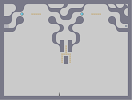Hover over the thumbnail for a full-size version.

Author krusch author:krusch less unrated 2014-12-16 2014-12-16 2 more votes required for a rating. \$Sender Geibel#krusch#less#000110100000000000000000071=010000000000000000879007=0000000000000000110;19000000000000000001=0:=0000000000000000009000000000000000000000000000000000000000000000000000000000000000000000000000000000000000000000000000000000000000000800000000000000000000006<0;<000000000000000000010:100;1<000000000000001806879068000000000000796<0:=0011100000000000=07100000000011000000000;1=0;<001110000000000001907968079000000000000010;100:1=00000000000007=0:=0000000000000000009000000000000000000000000000000000000000000000000000000000000000000000000000000000000000000000000000000000000000000800000000000000000000006<0;<000000000000000000810:1800000000000000000618006<00000000000000007111<010000000000000000:9611010000000000000000|5^357.485234583362,566!0^396,324!0^384,324!0^372,324!0^372,336!11^36,156,756,156!0^408,324!0^420,324!0^420,336!0^420,348!0^372,348!0^396,288!0^396,300!0^396,312!0^396,276!0^564,84!0^576,84!0^588,84!0^204,84!0^216,84!0^228,84!0^420,360!0^420,372!0^372,372!0^372,360!0^600,84!0^552,84!0^240,84!0^192,84!6^132,84,2,0,0,0!6^492,84,2,0,0,0# Groove is in the heart.

## Other maps by this author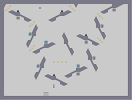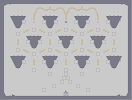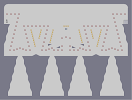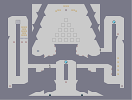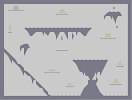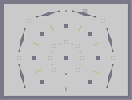acid roach Untitled Map Yoghurt Green Valentine (edit) learn2die Mea Culpa